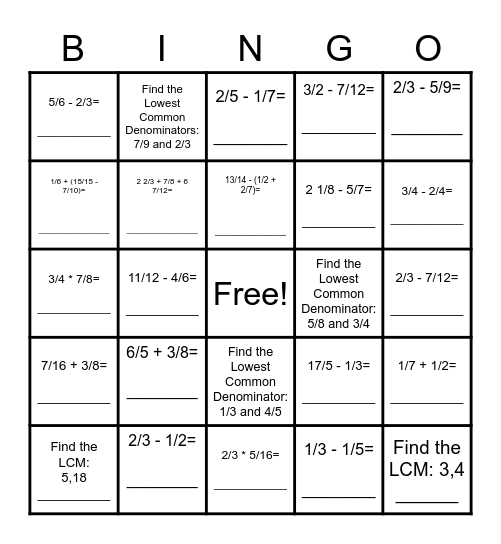# Fractions BingoThis bingo card has a free space and 44 words: 2/3 + 3/4= _____, Find the LCM: 3,4 ______, 4/5 - 3/5+ 2/7 = ________, Find the LCM: 5,18 __________, Find the LCM: 12, 13: __________, 1/3 - 1/5= ________, 2/5 - 1/7= ________, Find the Lowest Common Denominator: 2/5 and 1/6, 2/3 - 5/9= ________, 2/3 - 1/2= ________, 3/2 - 7/12= _________, Find the Lowest Common Denominator: 5/8 and 3/4, Find the Lowest Common Denominator: 1/3 and 4/5, 6/5 + 3/8= ________, 3/4 * 3/6= ___________, 2/3 * 5/16= ___________, 3/32 * 8/16= ___________, 3/4 * 7/8= ___________, Find the Lowest Common Denominators: 3/10 and 9/8, 3/4 - 2/4= ___________, Find the Lowest Common Denominators: 7/9 and 2/3, 4/5 + 6/7= ___________, 3/4 * 3/14= ___________, 5/6 - 2/3= ___________, 4/50 + 3/5= __________, 2/3 - 7/12= __________, 9/10 + 2/100= ____________, 4/9 + 1/4= __________, 17/5 - 1/3= __________, 7/16 + 3/8= __________, 2/5 + 1/4= __________, 1/7 + 1/2= __________, 1/2 - 3/16= __________, 7/8 - 2/3= __________, 11/12 - 4/6= __________, 7/18 + 5/9= __________, (7/8 + 1/12) - 1/2= __________, (11/18 - 4/9) + 1/6= __________, 13/14 - (1/2 + 2/7)= _______________, 1/6 + (15/15 - 7/10)= ________________, 2 1/8 - 5/7= __________, 12 1/3 - 2 1/4= ____________, 2 2/3 + 7/8 + 6 7/12= ________________ and 1 10/15 - 8/9= _____________.

## Play Online